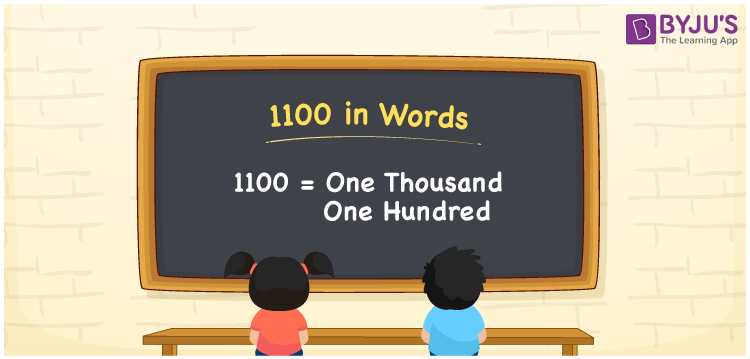# 1100 in Words

1100 in words is written as One thousand one hundred. In both the International System of Numerals and the Indian System of Numerals, 1100 is written as One thousand one hundred. The number 1100 is a Cardinal Number as it could represent some quantity. For example, “the dress costs 1100 rupees”.

 1100 in words One thousand one hundred One thousand one hundred in Numerical Form 1100

## 1100 in English Words

1100 in English words is read as “One thousand one hundred”.## How to Write 1100 in Words?

To write 1100 in words, we shall use the place value chart. In the place value chart, put 1 in the thousands, 1 in the hundreds, and 0 in the rest. Let us make a place value chart to write the number 1100 in words.

 Thousands Hundreds Tens Ones 1 1 0 0

Thus, we can write the expanded form as

1 × Thousand + 1 × Hundred + 0 × Ten + 0 × One

= 1 × 1000 + 1 × 100 + 0 × 10 + 0 × 1

= 1000 + 100 + 0 + 0

= 1100

= One thousand one hundred.

1100 is a natural number, the successor of 1099 and the predecessor of 1101.

1100 in words – One thousand one hundred

• Is 1100 an odd number? – No
• Is 1100 an even number? – Yes
• Is 1100 a perfect square number? – No
• Is 1100 a perfect cube number? – No
• Is 1100 a prime number? – No
• Is 1100 a composite number? – Yes

## Frequently Asked Questions on 1100 in Words

Q1

### How to write 1100 in words?

1100 in words can be written as One thousand one hundred.
Q2

### How to write 1100 in the International and Indian System of Numerals?

In both, the system of numerals, 1100 in words, is written as One thousand one hundred.
Q3

### How to write 1100 in a place value chart?

In the place value chart, write 1 in the thousands and the hundreds place and 0 in the tens and the ones place respectively.
Test your Knowledge on 1100 in Words